Select Page

# CBSE Maths 12 Science Vector Algebra MCQ Solutions in English

CBSE Maths 12 Science Vector Algebra MCQ Solutions in English to enable students to get Solutions in a narrative video format for the specific question.

Expert Teacher provides CBSE Maths 12 Science Vector Algebra MCQ Solutions through Video Solutions in English language. This video solution will be useful for students to understand how to write an answer in exam in order to score more marks. This teacher uses a narrative style for a question from Vector Algebra not only to explain the proper method of answering question, but deriving right answer too.

Please find the question below and view the Solution in a narrative video format.

Question:

Solution Video in English:

You can select video Solutions from other languages also. Please check Solutions in ( Hindi )

## Similar Questions from CBSE, 12th Science, Maths, Vector Algebra

Question 1 :  Write the value of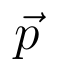for which the vectors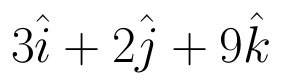and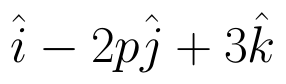are parallel vectors.  (View Answer Video)

Question 2 : Find the magnitude of two vectors a and b having the same magnitude and such that the angle between them is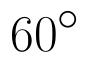and their scalar product is 1/2. (View Answer Video)

Question 3 : Find the angle between the vectors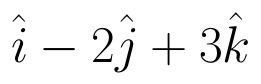and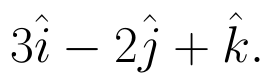(View Answer Video)

Question 4 : Find the unit vector in the direction of vector PQ, where P and Q are the points (1, 2, 3) and (4, 5, 6) respectively. (View Answer Video)

Question 5 : Find the position vector of a point which divides the join of points with position vectors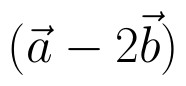and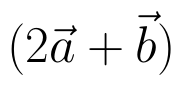externally in the ration 2:1.  (View Answer Video)

### Differential Equations

Question 1 : Write the degree of the differential equation :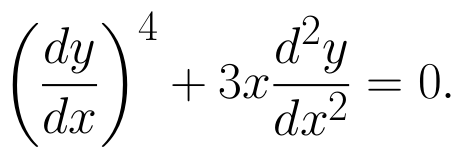(View Answer Video)

Question 2 : Find the particular solution of the differential equation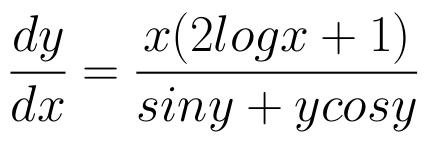given that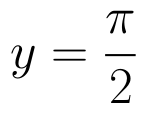where x = 1. (View Answer Video)

Question 3 : Find the particular solution of the differential equation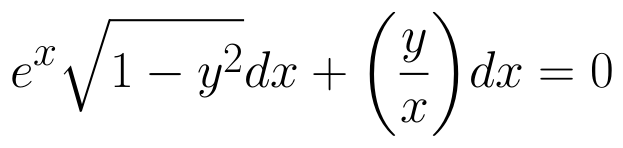given that y = 1 when x = 0. (View Answer Video)

Question 4 : Find the general solution of differential equation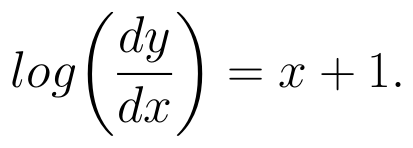(View Answer Video)

Question 5 : Solve the following differential equation :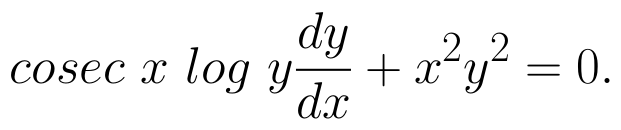(View Answer Video)

### Integrals

Question 1 : Find :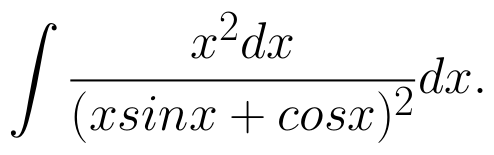(View Answer Video)

Question 2 : Evaluate :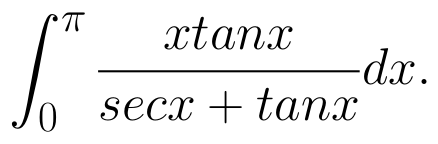(View Answer Video)

Question 3 : Find :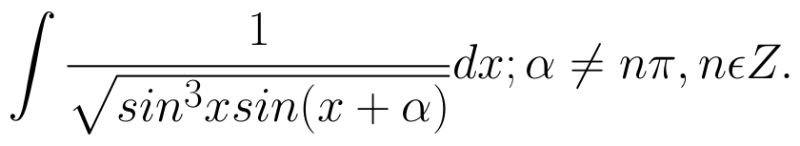(View Answer Video)

Question 4 : Find the integral of the function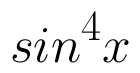. (View Answer Video)

Question 5 : Find :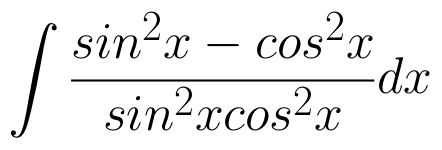(View Answer Video)

### Application of Derivatives

Question 1 : The normal at the point (1, 1) on the curve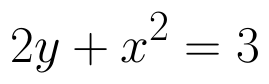is: (View Answer Video)

Question 2 : Find the maximum profit that a company can make if the profit function is.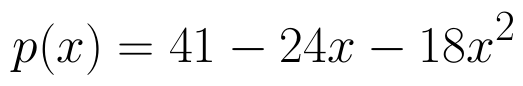(View Answer Video)

Question 3 : Find the approximate value of f(5.001) where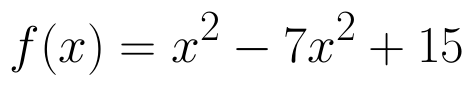. (View Answer Video)

Question 4 : The function f is a differentiable function and satisfies the functional equation f(x) + f(y) = f(x + y) – xy – 1for every pair x, y of real numbers. If f(1) = 1, then the number of integers n ≠ 1 for which f(n) = n is_______________. (View Answer Video)

Question 5 : The slope of the normal to the curve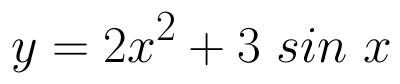at x = 0 is: (View Answer Video)